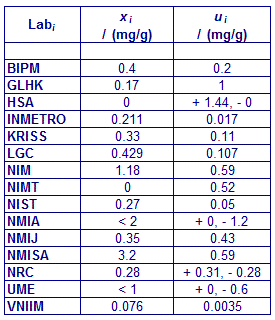Comparison
Comparison close
Results

CCQM-K55.d : Mass fraction of Folic acid and impurity assessment

MEASURAND : Mass fraction of Folic acid in CCQM-K55.d samples

Degrees of equivalence : offset Di and expanded uncertainty (k = 2) Ui expressed in mg/g, and also in relative terms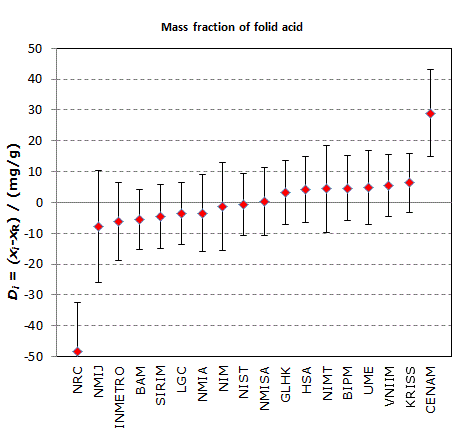CCQM-K55.d : Mass fraction of Folic acid and impurity assessment

MEASURAND : Mass fraction of total related structure impurity content in CCQM-K55.d samples

Degrees of equivalence : offset Di and expanded uncertainty (k = 2) Ui expressed in mg/g, and also in relative terms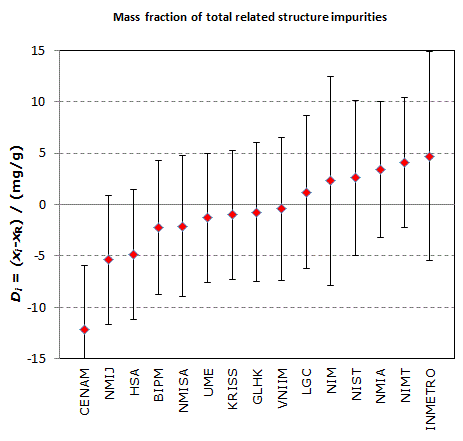CCQM-K55.d : Mass fraction of Folic acid and impurity assessment

MEASURAND : Mass fraction of Water in CCQM-K55.d samples

Degrees of equivalence : offset Di and expanded uncertainty (k = 2) Ui expressed in mg/g, and also in relative terms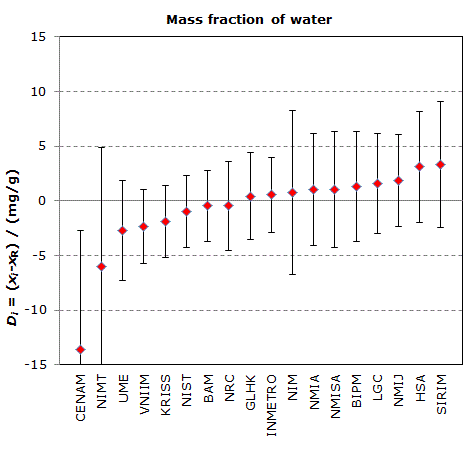CCQM-K55.d : Mass fraction of Folic acid and impurity assessment

MEASURAND : Mass fraction of Volatile organic content in CCQM-K55.d samples

Degrees of equivalence : offset Di and expanded uncertainty (k = 2) Ui expressed in mg/g, and also in relative terms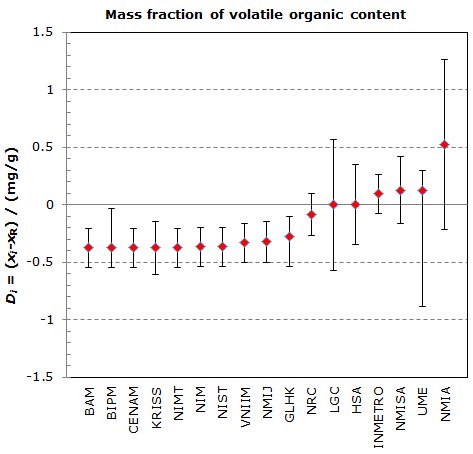CCQM-K55.d : Mass fraction of Folic acid and impurity assessment

MEASURAND : Mass fraction of Non-volatile content in CCQM-K55.d samples

Degrees of equivalence : offset Di and expanded uncertainty (k = 2) Ui expressed in mg/g, and also in relative terms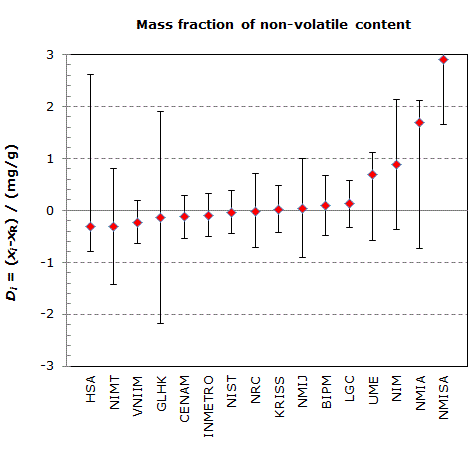Comparison
Comparison close
Results

CCQM-K55.d : Mass fraction of Folic acid and impurity assessment

MEASURAND : Mass fraction of Folic acid in CCQM-K55.d samples

Degrees of equivalence : offset Di and expanded uncertainty (k = 2) Ui expressed in mg/g

xR = 906.5 mg/g and 2 uR = 9.6 mg/g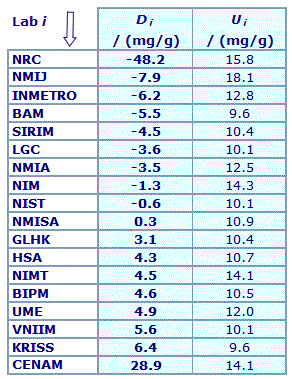Results are presented under A4 printable format in Summary Results (PDF file).

CCQM-K55.d : Mass fraction of Folic acid and impurity assessment

MEASURAND : Mass fraction of total related structure impurity content in CCQM-K55.d samples

Degrees of equivalence : offset Di and expanded uncertainty (k = 2) Ui expressed in mg/g

xR = 12.6 mg/g and 2 uR = 6.2 mg/g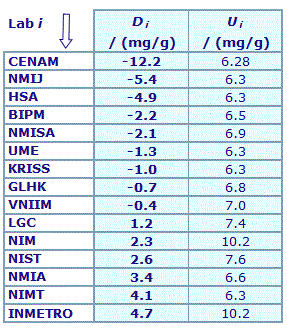Results are presented under A4 printable format in Summary Results (PDF file).

CCQM-K55.d : Mass fraction of Folic acid and impurity assessment

MEASURAND : Mass fraction of Water in CCQM-K55.d samples

Degrees of equivalence : offset Di and expanded uncertainty (k = 2) Ui expressed in mg/g

xR = 78.0 mg/g and 2 uR = 3.2 mg/g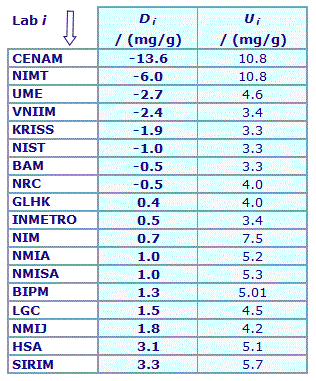Results are presented under A4 printable format in Summary Results (PDF file).

CCQM-K55.d : Mass fraction of Folic acid and impurity assessment

MEASURAND : Mass fraction of Volatile organic content in CCQM-K55.d samples

Degrees of equivalence : offset Di and expanded uncertainty (k = 2) Ui expressed in mg/g

xR = 0.374 mg/g and 2 uR = 0.166 mg/g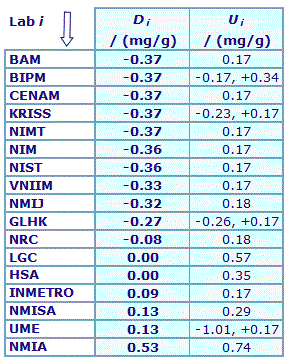Results are presented under A4 printable format in Summary Results (PDF file).

CCQM-K55.d : Mass fraction of Folic acid and impurity assessment

MEASURAND : Mass fraction of Non-volatile content in CCQM-K55.d samples

Degrees of equivalence : offset Di and expanded uncertainty (k = 2) Ui expressed in mg/g

xR = 0.3 mg/g and 2 uR = 0.4 mg/g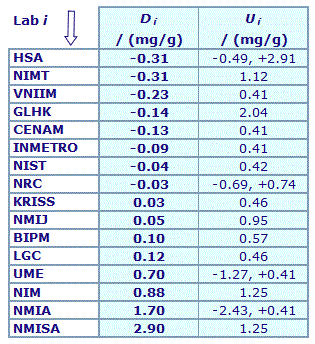Results are presented under A4 printable format in Summary Results (PDF file).

Comparison
Comparison close
CC comparison
 Metrology area, Sub-field Chemistry and Biology, Organics Description Purity assessment of high purity organic materials: Folic acid Time of measurements 2015 - 2016 Status Approved for equivalence
 Final Reports of the comparisons References Measurand Mass fraction in mg/g of Folic Acid in a sample. Nominal value in excess of 900 mg/g Required: Mass fraction content of main component Optional: Mass fraction content of impurities Transfer device Crimp-sealed amber glass vial containing 500 mg of the CCQM-K55.d comparison material as a crystalline powder.
 Comparison type Key Comparison Consultative Committee CCQM (Consultative Committee for Amount of Substance) Conducted by CCQM (Consultative Committee for Amount of Substance) Comments Results published on 14 May 2018 Purity assessment of high purity organic materials: Folic acid Pilot institute BIPM Bureau International des Poids et Mesures BIPM - International Organization Contact person Steven Westwood +33 (0) 1 45 07 70 57
First Name Last Name
wwww@ww.www +356719836 Institute 1 Institute 1 Khmelnitskiy
Pilot laboratory
BIPM

Bureau International des Poids et Mesures, BIPM - International Organization, N/A

 BAM Bundesanstalt für Materialforschung und -prüfung, Germany, EURAMET
 CENAM Centro Nacional de Metrologia, Mexico, SIM
 GL Government Laboratory, Hong Kong, China, APMP
 HSA Health Science Authority, Singapore, APMP
 INMETRO Instituto Nacional de Metrologia, Qualidade e Tecnologia, Brazil, SIM
 KRISS Korea Research Institute of Standards and Science, Korea, Republic of, APMP
 LGC Laboratory of the Government Chemist, United Kingdom, EURAMET
 NIM National Institute of Metrology, China, APMP
 NIMT National Institute of Metrology (Thailand), Thailand, APMP
 NIST National Institute of Standards and Technology, United States, SIM
 NMIA National Measurement Institute, Australia, Australia, APMP
 NMIJ AIST National Metrology Institute of Japan, Japan, APMP
 NMISA National Metrology Institute of South Africa, South Africa, AFRIMETS
 NRC National Research Council, Canada, SIM
 UME TÜBITAK Ulusal Metroloji Enstitüsü, Türkiye, EURAMET
 VNIIM D.I. Mendeleyev Institute for Metrology, Rosstandart, Russian Federation, COOMET
Comparison
Comparison close
Results

CCQM-K55.d : Mass fraction of Folic acid and impurity assessment

MEASURAND : Mass fraction of Folic acid in CCQM-K55.d samples

 The key comparison reference values , xR, and associated standard uncertainty (uR) were assigned for the mass fraction of the following impurity classes: total related structure impurity content, water, volatile organic content and non-volatile content. They are consensus values derived from the individual data supplied by each participant.  Folic acid:                      xR = 906.5 mg/g     uR = 4.8 mg/g Total impurity content:    xR = 12.6 mg/g      uR = 3.1 mg/g Water:                           xR = 78.0 mg/g      uR = 1.6 mg/g Volatile organic content:  xR = 0.374 mg/g    uR = 0.083 mg/g Non-volatile content:       xR = 0.3 mg/g        uR = 0.2 mg/g
 The degree of equivalence of laboratory i with respect to the key comparison reference value is given by a pair of terms: Di = (xi - xR) and Ui, its expanded uncertainty (k = 2), both expressed in mg/g, with Ui = 2(ui2 + uR2)1/2.

CCQM-K55.d : Mass fraction of Folic acid and impurity assessment

MEASURAND : Mass fraction of total related structure impurity content in CCQM-K55.d samples

 The key comparison reference values , xR, and associated standard uncertainty (uR) were assigned for the mass fraction of the following impurity classes: total related structure impurity content, water, volatile organic content and non-volatile content. They are consensus values derived from the individual data supplied by each participant.  Folic acid:                      xR = 906.5 mg/g     uR = 4.8 mg/g Total impurity content:    xR = 12.6 mg/g      uR = 3.1 mg/g Water:                           xR = 78.0 mg/g      uR = 1.6 mg/g Volatile organic content:  xR = 0.374 mg/g    uR = 0.083 mg/g Non-volatile content:       xR = 0.3 mg/g        uR = 0.2 mg/g
 The degree of equivalence of laboratory i with respect to the key comparison reference value is given by a pair of terms: Di = (xi - xR) and Ui, its expanded uncertainty (k = 2), both expressed in mg/g, with Ui = 2(ui2 + uR2)1/2.

CCQM-K55.d : Mass fraction of Folic acid and impurity assessment

MEASURAND : Mass fraction of Water in CCQM-K55.d samples

 The key comparison reference values , xR, and associated standard uncertainty (uR) were assigned for the mass fraction of the following impurity classes: total related structure impurity content, water, volatile organic content and non-volatile content. They are consensus values derived from the individual data supplied by each participant.  Folic acid:                      xR = 906.5 mg/g     uR = 4.8 mg/g Total impurity content:    xR = 12.6 mg/g      uR = 3.1 mg/g Water:                           xR = 78.0 mg/g      uR = 1.6 mg/g Volatile organic content:  xR = 0.374 mg/g    uR = 0.083 mg/g Non-volatile content:       xR = 0.3 mg/g        uR = 0.2 mg/g
 The degree of equivalence of laboratory i with respect to the key comparison reference value is given by a pair of terms: Di = (xi - xR) and Ui, its expanded uncertainty (k = 2), both expressed in mg/g, with Ui = 2(ui2 + uR2)1/2.

CCQM-K55.d : Mass fraction of Folic acid and impurity assessment

MEASURAND : Mass fraction of Volatile organic content in CCQM-K55.d samples

 The key comparison reference values , xR, and associated standard uncertainty (uR) were assigned for the mass fraction of the following impurity classes: total related structure impurity content, water, volatile organic content and non-volatile content. They are consensus values derived from the individual data supplied by each participant.  Folic acid:                      xR = 906.5 mg/g     uR = 4.8 mg/g Total impurity content:    xR = 12.6 mg/g      uR = 3.1 mg/g Water:                           xR = 78.0 mg/g      uR = 1.6 mg/g Volatile organic content:  xR = 0.374 mg/g    uR = 0.083 mg/g Non-volatile content:       xR = 0.3 mg/g        uR = 0.2 mg/g
 The degree of equivalence of laboratory i with respect to the key comparison reference value is given by a pair of terms: Di = (xi - xR) and Ui, its expanded uncertainty (k = 2), both expressed in mg/g, with Ui = 2(ui2 + uR2)1/2.

CCQM-K55.d : Mass fraction of Folic acid and impurity assessment

MEASURAND : Mass fraction of Non-volatile content in CCQM-K55.d samples

 The key comparison reference values , xR, and associated standard uncertainty (uR) were assigned for the mass fraction of the following impurity classes: total related structure impurity content, water, volatile organic content and non-volatile content. They are consensus values derived from the individual data supplied by each participant.  Folic acid:                      xR = 906.5 mg/g     uR = 4.8 mg/g Total impurity content:    xR = 12.6 mg/g      uR = 3.1 mg/g Water:                           xR = 78.0 mg/g      uR = 1.6 mg/g Volatile organic content:  xR = 0.374 mg/g    uR = 0.083 mg/g Non-volatile content:       xR = 0.3 mg/g        uR = 0.2 mg/g
 The degree of equivalence of laboratory i with respect to the key comparison reference value is given by a pair of terms: Di = (xi - xR) and Ui, its expanded uncertainty (k = 2), both expressed in mg/g, with Ui = 2(ui2 + uR2)1/2.
Comparison
Comparison close
Results

CCQM-K55.d : Mass fraction of Folic acid and impurity assessment

MEASURAND : Mass fraction of Folic acid in CCQM-K55.d samples

xi : result of measurement carried out by laboratory i
ui : combined standard uncertainty of xi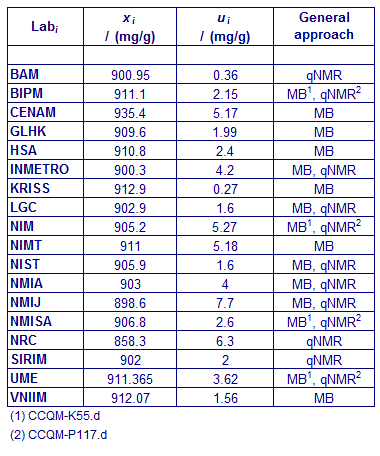CCQM-K55.d : Mass fraction of Folic acid and impurity assessment

MEASURAND : Mass fraction of total related structure impurity content in CCQM-K55.d samples

xi : result of measurement carried out by laboratory i
ui : combined standard uncertainty of xi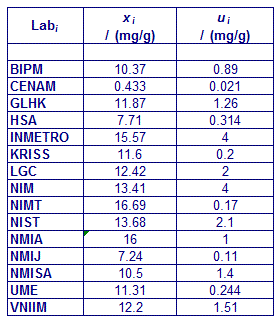CCQM-K55.d : Mass fraction of Folic acid and impurity assessment

MEASURAND : Mass fraction of Water in CCQM-K55.d samples

xi : result of measurement carried out by laboratory i
ui : combined standard uncertainty of xi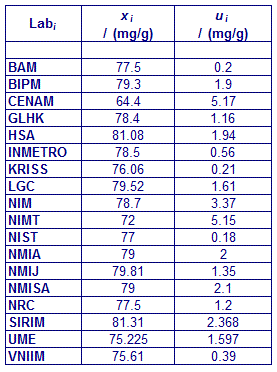CCQM-K55.d : Mass fraction of Folic acid and impurity assessment

MEASURAND : Mass fraction of Volatile organic content in CCQM-K55.d samples

xi : result of measurement carried out by laboratory i
ui : combined standard uncertainty of xi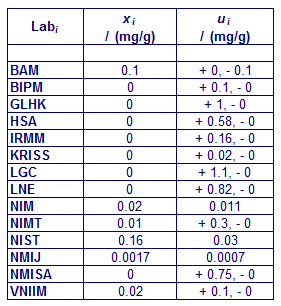CCQM-K55.d : Mass fraction of Folic acid and impurity assessment

MEASURAND : Mass fraction of Non-volatile content in CCQM-K55.d samples

xi : result of measurement carried out by laboratory i
ui : combined standard uncertainty of xi